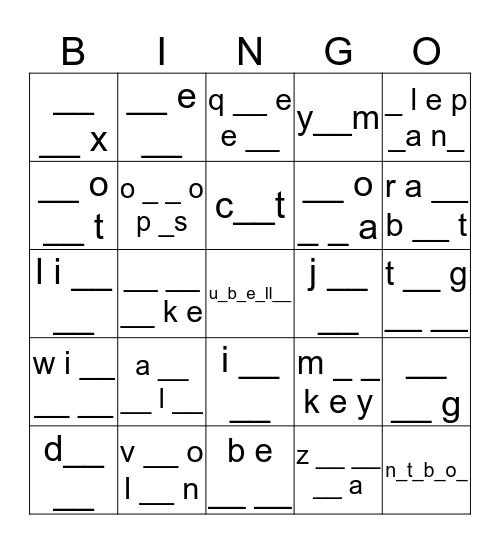# AlphabetThis bingo card has 25 words: a __ __ l __, b e __ __, c__t, d__ __, _ l e p _a n_, __ __ x, __ o __ t, __ e __, j __ __, i __ __, __ o _ _ a, l i __ __, m _ _ k e y, __ __ g, o _ _ o p _s, n_t_b_o_, t __ g __ __, q __ e e __, r a __ b __ t, __ __ __ k e, u_b_e_ll__, v __ o l __ n, w i __ __ __, y__m and z __ __ __ a.

## Play Online